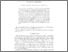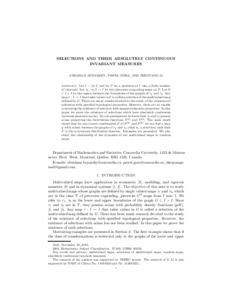Title:

# Selections and their absolutely continuous invariant measures

Boyarsky, Abraham, Góra, Paweł and Li, Zhenyang (2014) Selections and their absolutely continuous invariant measures. Journal of Mathematical Analysis and Applications, 413 (1). pp. 100-113. ISSN 0022247XPreview
Other (application/pdf)
Selections_November_2013_3.PDF - Accepted Version
234kB

Official URL: http://dx.doi.org/10.1016/j.jmaa.2013.11.043

## Abstract

Let $I=[0,1]$ and let $P$ be a partition of $I$ into a finite number of intervals. Let $\tau _{1},$ $\tau _{2}; I\rightarrow I$ be two piecewise expanding maps on $P.$ Let $G$ $\subset I\times I$ be the region between the
boundaries of the graphs of $\tau _{1}$ and $\tau _{2}.$ Any map $\tau :I\rightarrow I$ that takes values in $G$ is called a selection of the multivalued map defined by $G.$ There are many results devoted to the study of the existence of selections with specified topological properties.
measure-theoretic properties. In this paper we prove the existence of
selections which have absolutely continuous invariant measures (acim). By
our assumptions we know that $\tau _{1}$ and $\tau _{2}$ possess acims
preserving the distribution functions $F^{(1)}$ and $F^{(2)}.$
The main result shows that for any convex combination $F$ of $F^{(1)}$ and $% F^{(2)}$ we can find a map $\eta$ with values between the graphs of $\tau _{1}$ and $\tau _{2}$ (that is, a selection) such that $F$ is the $\eta$%
-invariant distribution function. Examples are presented. We also study the
relationship of the dynamics of our multivalued maps to random maps.

Divisions: Concordia University > Faculty of Arts and Science > Mathematics and Statistics Article Yes Boyarsky, Abraham and Góra, Paweł and Li, Zhenyang Journal of Mathematical Analysis and Applications 2014 10.1016/j.jmaa.2013.11.043 980102 PAWEL GORA 25 Jun 2015 16:03 18 Jan 2018 17:50
All items in Spectrum are protected by copyright, with all rights reserved. The use of items is governed by Spectrum's terms of access.

Repository Staff Only: item control pageResearch related to the current document (at the CORE website)
Back to top